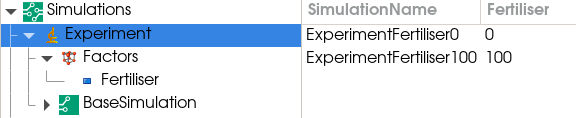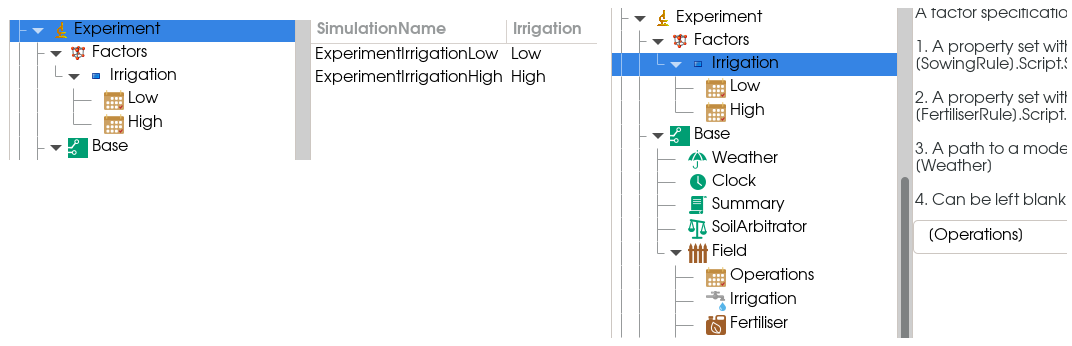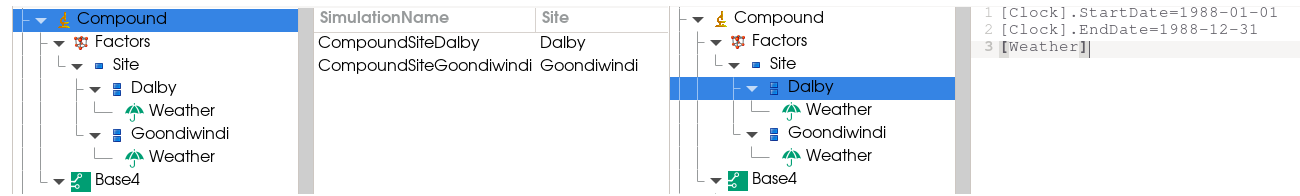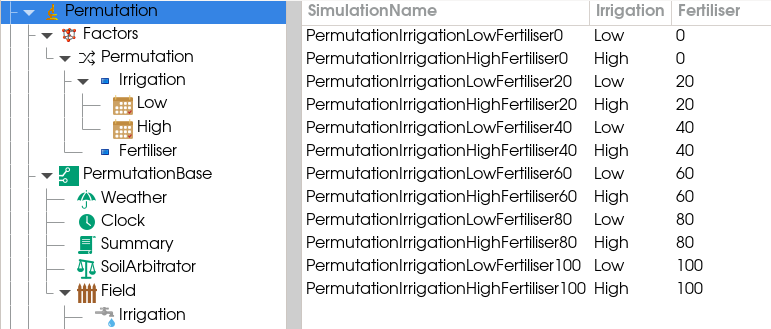# Factorial Simulations

An factorial allows a single simulation to be run multiple times with different parameters or inputs. At its most simple, a factorial consists of an experiment node with two children - a simulation, and a factors node. The simulation (sometimes called the base simulation) defines the default behaviour of the experiment. The factors node should contain multiple factor children, where each factor defines one or more treatments (levels) of the experiment.When run, the experiment will generate one simulation for each factor level. The factors and factor levels are defined by the factor configurations under the Factors node. There are different types of factors, and they can be combined as needed. The different types of factors are described below, and examples of each type of factor are given in the factorial example file (Factorial.apsimx) which is included with APSIM installations.

## Factor

The factor node allows a single model or property to be modified. The factor’s behaviour is defined by the factor specification, which is a piece of text. The factor specification can be one of:

• A property set with multiple values, separated by commas e.g.

`[SowingRule].Script.SowingDate = 2000-11-01, 2000-12-03`

This will result in one treatment being generated for each property value.

• A property set with a range e.g.

`[FertiliserRule].Script.ApplicationAmount = 0 to 200 step 20`

This will result in one treatment being generated for each property value.

• A path to a model that will be replaced with one or more children of this factor that have a matching type e.g.• Can be empty if the factor has one or more composite factor children. In this case, one treatment will be generated for each composite factor child.

## Composite Factor

The composite factor allows multiple models or properties to be changed in a single treatment. The composite factor user interface is a freeform (multiline) text input. Each line should contain a single factor specification string, which can be any valid factor specification (described above). If changing a property, only one value is allowed per line (ie no comma separated values).## Permutations

The permutations node should have multiple factor children, and it will generate one factor level (treatment) for each permutation of its child factors’ values.